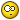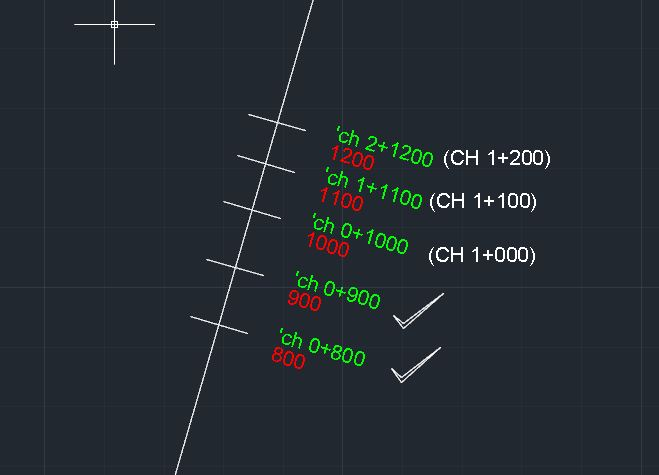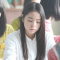Lisp Problem. Very new to Lisp 'Chainage Line'Recommended PostsHi,

I found this online and it works. But the out put is different to what the Engineer/Client is looking for.

Chainage prints out at '100', '200' -----> '1100', '1200' etc.

Im looking for Chainage to print out 'ch 0+100', ' ch 0+200' -----> 'ch 1+100', 'ch 1+200'

Any help would be great even to point me in the wright direction on how to do this. I have attached the Lisp which works here.

(defun c:CHAINTXT ( / ) (Chainage_text))

(defun Chainage_text (/)

(setq ss (ssget)

count 0

dist 100.0

offset 5

height 2.5

)

(repeat (sslength ss)

(setq ent (ssname ss count)

obj (vlax-ename->vla-object ent)

chainage dist

)

(while (and

(setq point1 (vlax-curve-getPointAtDist obj chainage))

(setq point2 (vlax-curve-getPointAtDist obj (+ chainage 0.1)))

)

(setq bearing (+ (angle point1 point2) (/ PI 2.0)))

(entmake (append

'((0 . "LINE") (100 . "AcDbEntity") (67 . 0) (410 . "Model") (8 . "0") (100 . "AcDbLine"))

(list (cons 10 (polar point1 bearing offset)))

(list (cons 11 (polar point1 (+ bearing PI) offset)))

'((210 0.0 0.0 1.0))

)

)

(entmake (append

'((0 . "TEXT") (100 . "AcDbEntity") (67 . 0) (410 . "Model") (8 . "0") (100 . "AcDbText"))

(list (cons 10 (polar point1 (+ bearing PI) (* offset 2.0))))

(list (cons 40 height))

(list (cons 1 (rtos chainage 2 3)))

(list (cons 50 (+ bearing PI)))

'((41 . 1.0) (51 . 0.0) (7 . "Standard") (71 . 0) (72 . 0) (11 0.0 0.0 0.0) (210 0.0 0.0 1.0) (100 . "AcDbText") (73 . 0))

)

)

(setq chainage (+ chainage dist))

)

(setq count (1+ count))

)

)

Share on other sitesSomething like this ?

(defun c:Test (/ bearing chainage dist ent height obj offset point1
point2 ss i n
)
;; Tharwat 17.12.2014 ;;
(setq dist 100.0
offset 5
height 2.5
)
(if (setq ss (ssget '((0 . "LWPOLYLINE,SPLINE"))))
(repeat (setq i (sslength ss))
(setq ent      (ssname ss (setq i (1- i)))
obj      (vlax-ename->vla-object ent)
chainage dist
n 0
)
(while
(and
(setq point1 (vlax-curve-getPointAtDist obj chainage))
(setq point2 (vlax-curve-getPointAtDist obj (+ chainage 0.1)))
)
(setq bearing (+ (angle point1 point2) (/ pi 2.0)))
(entmake
(list '(0 . "LINE")
'(8 . "0")
(cons 10 (polar point1 bearing offset))
(cons 11 (polar point1 (+ bearing pi) offset))
'(210 0.0 0.0 1.0)
)
)
(entmake
(list '(0 . "TEXT")
'(8 . "0")
(cons 10 (polar point1 (+ bearing pi) (* offset 2.0)))
(cons 40 height)
(cons 1
(strcat "'ch "
(if (> chainage 1000.)
(itoa (setq n (1+ n)))
"0"
)
"+"
(rtos chainage 2 3)
)
)
(cons 50 (+ bearing pi))
'(41 . 1.0)
'(51 . 0.0)
'(7 . "Standard")
'(71 . 0)
'(72 . 0)
'(11 0.0 0.0 0.0)
'(210 0.0 0.0 1.0)
'(73 . 0)
)
)
(setq chainage (+ chainage dist)
)
)
)
)
(princ)
)

Edited by Tharwat
Share on other sitesThanks Tharwat,

Its close to what I am looking for but i need the '0' beside the 'Ch 0+' to increment every time the chainage is greater than 1000 so it prints out

'Ch 0+500' for 500

'Ch 1+200' for 1200

'Ch 2+300' for 2300

Thanks for the help this could lead me on to getting it.

Share on other sitesI modified the program above , try it and let me know .

Share on other sitesThanks Tharwat

Getting there alright

That is the idea of what Im looking for. I think after each increment of the 1000 the count will need to be reset to = 0 as the Ch 1, counts for every 100m chainage along the line. after it reaches 1000 so for

chainage 1000 its Ch 1+000

Chainage 1100 its Ch 2+1100 ( where I am looking for "Ch 1+100" for Chainage 1100)

Hopefully there is a way of doing this or I am probably asking too much:?Thanks again.

Share on other sitesNot that clear enough , but try the modification again .

Share on other siteshi Tharwat, i think OP a civil guy? Try this formatting function by ymg. i also borrow it for my station label routine;;http://www.theswamp.org/index.php?topic=45311.0
;; rtosta                     by ymg  September 2013                          ;
;;                                                                            ;
;; Arguments:   sta Real number to format as a Station                        ;
;;             unit 1 for Imperials,                                          ;
;;                  2 for Metrics.                                            ;
;;             prec Integer for number of decimals                            ;
;;                                                                            ;
;; Examples: (rtosta 0 1 0)  -> "0+00"   (rtosta 1328.325 1 2) -> "13+28.33"  ;
;;           (rtosta 0 2 0)  -> "0+000"  (rtosta 1328.325 2 2) -> "1+328.33"  ;
;;                                                                            ;
;; If sta is negative, format is as follow:                                   ;
;;                                       (rtosta -1328.325 1 2) -> "13-28.33" ;
;;                                       (rtosta -1328.325 2 2) -> "1-328.33" ;
;;                                                                            ;

(defun rtosta (sta unit prec / str a b dz)
(setq dz (getvar 'dimzin))
(setvar 'dimzin 0)
(setq str (rtos (abs sta) 2 prec))
(setvar 'dimzin dz)
(while (< (strlen str)
(if	(= prec 0)
(+ unit 2)
(+ prec (+ unit 3))
) ;_ end of if
) ;_ end of <
(setq str (strcat "0" str))
) ;_ end of while
(setq	a (if (= prec 0)
(- (strlen str) unit)
(- (strlen str) prec (+ unit 1))
) ;_ end of if
b (substr str 1 (- a 1))
a (substr str a)
) ;_ end of setq
(strcat b
(if (minusp sta)
"-"
"+"
) ;_ end of if
a
) ;_ end of strcat
) ;_ end of defun

Share on other sitesIdeally it would work like the white text to be offset from the line. the red is the original lisp and green is what you have helped me with.

If i could get this it would be a great help for alot of projects im working on

Share on other sitesPlease read the Code Posting Guidelines and edit your post to include the Code in Code Tags.

Share on other siteshi Tharwat, i think OP a civil guy?

Hi hanhphuc , Yeah ... you could be right , and I am actually mechanical guy@ OP. What's if the number is greater than 2000 ? how it should look like ?

Share on other sitesyes a Civil guy and appreciating the help here.

If greater than 2000 it would read "ch 2+000" and then "2+100" if greater than 3000 "ch 3+000" and "ch 3+100"

i have a small bit of programming from an Electronics back round and some how ended up working in Civil. and have no idea about lisp trying to get into it.

the if statment you added to help me is great but if i could get the count back to '0' and hopefully increment the Ch #+ everytime the count goes over 999.

Share on other sitesI think this one should be as you have requested , try it and let me know .

(defun c:Test (/ bearing chainage dist ent height obj offset point1
point2 ss i
)
;;	Tharwat 17.Dec.2014		;;
(setq dist 100.0
offset 5
height 2.5
)
(if (setq ss (ssget '((0 . "LWPOLYLINE"))))
(repeat (setq i (sslength ss))
(setq ent      (ssname ss (setq i (1- i)))
obj      (vlax-ename->vla-object ent)
chainage dist
)
(while
(and
(setq point1 (vlax-curve-getPointAtDist obj chainage))
(setq point2 (vlax-curve-getPointAtDist obj (+ chainage 0.1)))
)
(setq bearing (+ (angle point1 point2) (/ pi 2.0)))
(entmake
(list '(0 . "LINE")
'(8 . "0")
(cons 10 (polar point1 bearing offset))
(cons 11 (polar point1 (+ bearing pi) offset))
'(210 0.0 0.0 1.0)
)
)
(entmake
(list
'(0 . "TEXT")
'(8 . "0")
(cons 10 (polar point1 (+ bearing pi) (* offset 2.0)))
(cons 40 height)
(cons
1
(strcat "'ch "
(if (>= chainage 1000.)
(strcat (substr (rtos chainage 2 3) 1 1)
"+"
(substr (rtos chainage 2 3) 2)
)
(strcat "0"
"+"
(rtos chainage 2 3)
)
)
)
)
(cons 50 (+ bearing pi))
'(41 . 1.0)
'(51 . 0.0)
'(7 . "Standard")
'(71 . 0)
'(72 . 0)
'(11 0.0 0.0 0.0)
'(210 0.0 0.0 1.0)
'(73 . 0)
)
)
(setq chainage (+ chainage dist)
)
)
)
)
(princ)
)

Share on other sitesAwesome Tharwat.

Sorry for dragging that out. its my first time looking for anything like this .Hoping to get to a stage where I can help some one in the future.

Thanks again.

Share on other sitesAwesome Tharwat.

Sorry for dragging that out. its my first time looking for anything like this .Hoping to get to a stage where I can help some one in the future.

Thanks again.

Excellent , I am happy to hear thatYou are welcome anytime .

Share on other sitesI have one snag, when the distance on a given line is greater than '10,000' it prints for example 13200 it prints 'ch 1+3200' rather than 'ch 13+200'

I will try to fix this. as i said I am trying to teach myself. I know this is a formula issue and will get back if I have difficulties

Thanks again.

Share on other sitesI have one snag, when the distance on a given line is greater than '10,000' it prints for example 13200 it prints 'ch 1+3200' rather than 'ch 13+200'

I will try to fix this. as i said I am trying to teach myself. I know this is a formula issue and will get back if I have difficulties

Thanks again.

Sorry for the confusion .

Have a play with this modified program and let me know .

(defun c:Test (/ _output:value bearing chainage dist ent height obj
offset point1 point2 ss i
)
;;	Tharwat 17.Dec.2014		;;
(setq dist 100.0
offset 5
height 2.5
)
(defun _output:value (v / s)
(if (eq v 1000.)
(strcat (substr (rtos v 2 3) 1 1)
"+"
(substr (rtos v 2 3) 2)
)
(strcat (substr (setq s (rtos v 2 3)) 1 (- (strlen s) 3))
"+"
(substr (rtos v 2 3) (- (strlen s) 2))
)
)
)
(if (setq ss (ssget '((0 . "LWPOLYLINE"))))
(repeat (setq i (sslength ss))
(setq ent      (ssname ss (setq i (1- i)))
obj      (vlax-ename->vla-object ent)
chainage dist
)
(while
(and
(setq point1 (vlax-curve-getPointAtDist obj chainage))
(setq point2 (vlax-curve-getPointAtDist obj (+ chainage 0.1)))
)
(setq bearing (+ (angle point1 point2) (/ pi 2.0)))
(entmake
(list '(0 . "LINE")
'(8 . "0")
(cons 10 (polar point1 bearing offset))
(cons 11 (polar point1 (+ bearing pi) offset))
'(210 0.0 0.0 1.0)
)
)
(entmake
(list
'(0 . "TEXT")
'(8 . "0")
(cons 10 (polar point1 (+ bearing pi) (* offset 2.0)))
(cons 40 height)
(cons
1
(strcat "'ch "
(cond ((< chainage 1000.)
(strcat
"0"
"+"
(rtos chainage 2 3)
)
)
(t (_output:value chainage))
)
)
)
(cons 50 (+ bearing pi))
'(41 . 1.0)
'(51 . 0.0)
'(7 . "Standard")
'(71 . 0)
'(72 . 0)
'(11 0.0 0.0 0.0)
'(210 0.0 0.0 1.0)
'(73 . 0)
)
)
(setq chainage (+ chainage dist)
)
)
)
)
(princ)
)

Share on other sitesPlease read the Code Posting Guidelines and edit your post to include the Code in Code Tags.

............................

Share on other sitesHi dweafer , Do you understand what the meaning of the super moderator ?

Join the conversation

You can post now and register later. If you have an account, sign in now to post with your account.
Note: Your post will require moderator approval before it will be visible.×   Pasted as rich text.   Restore formatting

Only 75 emoji are allowed.